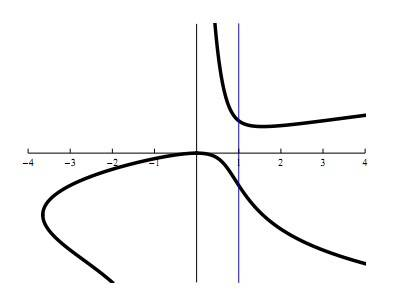# Find dy/dx of x^2 − (y^2) x = (3x − 3)yx^2−(y^2)x = (3x − 3)y

Using this graph and the equation I need to find two things.

(a) Formula which gives the slope dy/dx at every point (x, y) on the graph.
(b) As you can see in the picture, there are two points on the graph which have x-coordinate equal to 1. What are the exact slopes of the tangent lines at those two points?

I believe I correctly figured out (a) to be y'(x)= (2x-y^2-3y)/(2xy+3x-3)

However, I am not sure how to use this formula to find two different slopes for one value of x.

CompuChip
Homework Helper
I believe I correctly figured out (a) to be y'(x)= (2x-y^2-3y)/(2xy+3x-3)
That looks correct

However, I am not sure how to use this formula to find two different slopes for one value of x.
You are given the value of x... what else do you need to calculate the slope at a point?

HallsofIvy